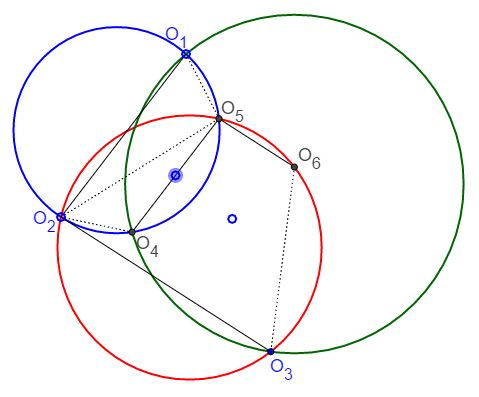# 3 Isosceles Trapezoids What Is It About? A Mathematical Droodle

Let there be 6 points O1, O2, O3, O4, O5 and O6. Assume also that in the hexagon O1O2O3O4O5O6 there are three pairs of parallel sides: O1O2 || O4O5, O2O3 || O5O6 and O3O4 || O6O1 and that each pair of parallel sides defines an isosceles trapezoid. The vertices of each of the trapezoids lie on a circle. What the applet is supposed to suggest is that if two of the circles coincide, then the third circle coincides with the other two.

(Due to this statement, it is impossible to have all three trapezoids isosceles unless all three circles coincide. The applet, therefore, insures that the red and the blue quadrilaterals are isosceles trapezoids. The green quadrilateral acquires that symmetry if and only if the three circles coincide.)### Lemma

Let each side of the hexagon O1O2O3O4O5O6 be parallel to its opposite mate. Then the hexagon is inscribable in a circle if and only if the three pairs of opposite sides define isosceles trapezoids.

### Proof (1)

First, assume that the three quadrilaterals O1O2O4O5, O2O3O5O6 and O3O4O6O1 are all isosceles trapezoids. The circumcenter of the first lies on the common perpendicular bisector of O1O2 and O4O5. The circumcenter of O2O3O5O6 lies on the common perpendicular bisector of O2O3 and O5O6. Consider the point S of intersection of the two lines. I wish to show that all six points are equidistant from S.

By construction, points O1 and O2 certainly are (from the first trapezoid) as are points O2 and O3 (from the second trapezoid). Also by construction, SO4 = SO5 and also SO5 = SO6.

In the third trapezoid, O3O4O6O1, we therefore have

SO1 = SO3 and SO4 = SO6

so that the perpendicular bisectors of the sides O1O3 and O4O6 cross at S, and, because of the symmetry of the isosceles trapezoid O3O4O6O1, the common perpendicular bisector of its bases, O3O4 and O6O1, also passes through S.

This completes the "if" portion of the proof. The "only if" portion is obvious. If a hexagon inscribed in a circle has the sides parallel to their opposites, then the opposite sides do of course define isosceles trapezoids.

### Proof (2)

The sides of an isosceles trapezoid are equal and so are its diagonals. Any two of the three trapezoids at hand share a diagonal, such that in all there are just three segments: O1O4, O2O5, and O3O6. Any two of the segments serve as diagonals of one of the three trapezoids and are, therefore, equal. Which implies that all three are equal. Having established that, we are now in a position to apply Problem 109 from [Shklyarsky]:

Prove that, if in a hexagon the opposite sides are parallel and the three diagonals joining the opposite vertices are equal, then the hexagon is inscribable in a circle.

A trapezoid with equal diagonals is necessarily isosceles. The common perpendicular bisector of O1O2 and O4O5 serves as the axis of symmetry of the trapezoid O1O2O4O5. It passes through the point of intersection of the diagonals O1O4 (or their extensions) and O2O5 and bisects the angle formed by the two. Similar conclusions could be drawn regarding the other two trapezoids. The three diagonals form a triangle in which the axes of symmetry of the three trapezoids bisect internal angles. The axes of symmetry are concurrent at the incenter of the triangle formed by the three diagonals. Naturally, all six points O1, ..., O6 are equidistant from that point.

### References

1. Floor van Lamoen, Private communication, May 2002.
2. D. O. Shklyarsky, N. N. Chentsov, I. M. Yaglom, Selected Problems and Theorems of Elementary Mathematics, v 2, Moscow, 1952